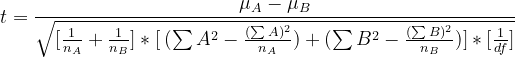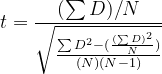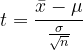Skip to content
Related Articles
T-test
• Last Updated : 27 May, 2021

Prerequisites – Hypothesis Testing, p-value

A t-test is a type of inferential statistic used to determine if there is a significant difference between the means of two groups, which may be related in certain features.If t-value is large => the two groups belong to different groups.
If t-value is small => the two groups belong to same group.

Terminologies involved

• Degree of freedom (df) – It tells us the number of independent variables used for calculating the estimate between 2 sample groups.[Eq-2]where,
df = degree of freedom
nS = size of the sample S

Suppose, we have 2 samples A and B. The df would be calculated as

df = (nA-1) + (nB -1)

• Significance level (α) – It is the probability of rejecting the null hypothesis when it is true. In simpler terms, it tells us about the percentage of risk involved in saying that a difference exist between two groups, when in reality it does not.

There are three types of t-tests, and they are categorized as dependent and independent t-tests.

1. Independent samples t-test: compares the means for two groups.
2. Paired sample t-test: compares means from the same group at different times (say, one year apart).
3. One sample t-test test: the mean of a single group against a known mean.

1. Independent sample t-test

Independent sample t-test, commonly known as unpaired sample t-test is used to find out if the differences found between two group is actually significant or just a random occurrence.

We can use this when:

• the population mean or standard deviation is unknown. (information about population is unknown)
• the two samples are separate/independent. For eg. boys and girls (the two are independent of each other)

Formula used:Eq-3

where,
t = t-value
A = Sample of A
B = Sample of B
μA = Mean of sample A
μB = Mean of sample B
nA = samele size of A
nB = sample size of B
df = degree of freedom

Steps involved

Step 1 - Find the sum of all values in each sample.
Step 2 - Square the sum values found in step 1.
Step 3 - Find the sum of square of individual values in each sample.
Step 4 - Calculate the mean of each sample.
Step 5 - Find the degree of freedom (df) using Eq-2.
Step 6 - Insert all the values found in Steps 1-4 into Eq-3 and find the calculated t-value.
Step 7 - Use the values of df and α (take α = 0.05 if not given) in the two-tails t-table (Click here) to
find the table value of t.
Step 8 - Compare values of t found in Step-6 and Step-7.

Interpreting the results

If tcal > ttable => p < (α=0.05) => significant difference between two groups found.
If tcal < ttable => p > (α=0.05) => no significant difference between two groups.

Example Problem (Step by Step)

Suppose, two independent sample data A and B is given, with the following values. We have to perform the Independent samples t-test for this data.

Step 1 -
∑A = 1 + 2 + 4 + 4 + 5 + 5 + 6 + 7 + 8 + 8 = 50
∑B = 1 + 2 + 2 + 3 + 3 + 4 + 5 + 6 + 7 + 7 = 40
Step 2 -
(∑A)2 = (50)2 = 2500
(∑B)2 =    (40)2 = 1600
Step 3 -
∑A2 = 12 + 22 + 42 + 42 + 52 + 52 + 62 + 72 + 82 + 82 = 300
∑B2 = 12 + 22 + 22 + 32 + 32 + 42 + 52 + 62 + 72 + 72 = 202
Step 4 -
n = 10
μA = (∑A / n) = 50/10 = 5
μB = (∑B / n) = 40/10 = 4
Step 5 -
df = (nA - 1) + (nB - 1) = (10-1) + (10-1) = 18 [using Eq-2]
Step 6 - Putting values found in Eq-3 to find the calculated value of t.
we get, tcal = 0.99
Step 7 - Let value of α = 0.05 and df = 18. Looking up the two-tailed t-table.
(See table below or refer link above)
we get, ttable = 2.10
Step 8 -
0.99 < 2.10 (tcal < ttable by 1.11)
=> no significant difference found between two groups.

2. Paired sample t-test

Paired sample t-test, commonly known as dependent sample t-test is used to find out if the difference in the mean of two samples is 0. The test is done on dependent samples, usually focusing on a particular group of people or thing. In this, each entity is measured twice, resulting in a pair of observations.

We can use this when:

• Two similar (twin like) samples are given. [Eg, Scores obtained in English and Math (both subjects)]
• The dependent variable (data) is continuous.
• The observations are independent of one another.
• The dependent variable is approximately normally distributed.

Formula UsedEq-4

where,
t = t-value
D = difference between the two samples (A-B)
N = sample size (same as n)

Steps Involved

Step 1 - Find the sum of differnce of each two samples in data. [∑D = ∑(A-B)]
Step 2 - Find the sum of square of each D found in Step 1. [(∑D2)]
Step 3 - Find the square of summation of D. [(∑D)2]
Step 4 - Put the values found from Steps 1-3 in Eq-4 and find the t-value.
Step 5 - Find the degree of freedom (df) using Eq-2.

NOTE :  Here, df is calculated as a whole for the data, not for each individual sample set. This is because the two samples A and B are twin like. (similar)

So, df = ∑(nS – 1) = N-1

Step 6 - Use the values of df and α (take α = 0.05 if not given) in the two-tails t-table (Click here) to
find the table value of t.
Step 7 - Compare values of t found in Step-4 and Step-6.

Interpretation of Results

Same as that of Independent samples t-test.

Example Problem (Step by Step)

Consider the following example. Scores (out of 25) of the subjects Math and SST are taken for a sample of 10 students. We have to perform the paired sample t-test for this data.

Step 1 and Step 2 - as shown in table above.
Step 3 - (∑D)2 = (71)2 = 5041
Step 4 - Putting values in Eq-4, we get
tcal = -4.96
Step 5 - df = n -1 = 10 - 1 = 9
Step 6 - Using df = 9 and α = 0.05 in table. We get,
ttable = 2.26
Step 7 - -4.96 < 2.26 (tcal < ttable by 7.22)
=> no significant difference found between two groups.

3. One sample t-test

One sample t-test is one of the widely used t-tests for comparison of the sample mean of the data to a particular given value. Used for comparing the sample mean to the true/population mean.

We can use this when:

the sample size is small. (under 30) data is collected randomly. data is approximately normally distributed.

Formula used:Eq-5

where,
t = t-value
x_bar = sample mean
μ = true/population mean
σ = standard deviation
n = sample size

Steps involved

Step 1 - Define the null (h0) and alternative (h1) hypothesis.
Step 2 - Calculate sample mean. (if not given)
[population mean, standard deviation, n is given]
Step 3 - Put the values found in Step 1 into Eq-5 and calculate t-value. (tcal)
Step 4 - Calculate degree of freedom (df). (same as done in paired sample t-test)
Step 5 - Take α = 0.05 if not given. Use the value of df and α and find ttable from one tailed t-table. (Click here)
Step 6 - Compare values of t found in Step-3 and Step-5.

Interpretation of Results

Same as that of Independent samples t-test.

Example Problem (Step by Step)

Consider the following example. The weights of 25 obese people were taken before enrolling them into the nutrition camp. The population mean weight is found to be 45 kg before starting the camp. After finishing the camp, for the same 25 people, the sample mean was found to be 75 with a standard deviation of 25. Did the fitness camp work?

Step 1 - h0 -> μ = 45 (sample mean is true mean)
h1 -> μ ≠ 45 (sample mean is not true mean)
Step 2 - Given,
x_bar = 75
μ = 45
σ = 25
n = 25
Step 3 - Putting the values from Step 2 in Eq-5. we get,
tcal = 6
Step 4 - df = n - 1 = 24
Step 5 - Using df = 24 and α = 0.05 in table. We get,
ttable = 1.711
Step 6 - 6 > 1.711 (tcal > ttable)
=> significant difference found between two groups.
=> the nutrition camp significantly impacted the weights and it was a success. 

The above discussed types of t-tests are widely used in the fields of research in hospitals by experts to gain important information about the medical data given to them about effects of various medicines and drugs on the population and helps them draw out important inferences regarding the same. However, it is the responsibility of the person to see to it that which t-test would bring out the best results and that all the assumptions of that t-test are adhered to. For any doubt/query, comment below.My Personal Notes arrow_drop_up